# Error Detection & Correction: Its Types Notes | Study Computer Networks - Computer Science Engineering (CSE)

## Computer Science Engineering (CSE): Error Detection & Correction: Its Types Notes | Study Computer Networks - Computer Science Engineering (CSE)

The document Error Detection & Correction: Its Types Notes | Study Computer Networks - Computer Science Engineering (CSE) is a part of the Computer Science Engineering (CSE) Course Computer Networks.
All you need of Computer Science Engineering (CSE) at this link: Computer Science Engineering (CSE)

Error Detection
For reliable communicati on errors must be detected and corrected. For error detection we are using many mechanisms.

Redundancy
One error detection mechanism is sending every data unit twice. The receiving device then would be able to do a bit for bit comparison between the two versions of the data. Any discrepancy would indicate an er ror, and an appropriate correction mechanism could be used.But instead of repeating the entire data stream, a shorter group of bits may be appended to the end of each unit. This technique is called redundancy because extra bits are redundant to the information. They are discarded as soon as the accuracy of the transm ission has been determined.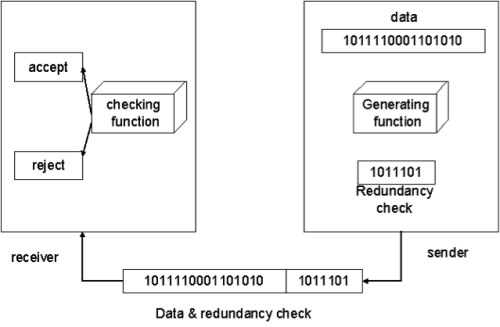Types
Four types of redundancy checks are used in data communications. They are,
1.     vertical redundancy check (VRC)
2.     longitudinal r edundancy check (LRC)
3.     cyclic redund ancy check (CRC)
4.     checksum

Vertical Redundancy Check:
It is also known as parity check. In this technique a redundant bit called a parity bit is appended to every data unit so that the total number of 1s in the unit includi ng the parity bit becomes even for even parity or odd for odd parity.
In even parity, the data unit is passed through the even parity generator. It counts the number of 1s in the data unit. If odd number of 1s, then it sets 1 in the parity bit to make the number of 1s as even. If the data unit having even number of 1s then it sets in the parity bit to maintain the number of 1s as even. When it reaches its destination, the receiver puts all bits through an even parity checking function. If it counts even number of 1s than there is no error. Otherwise there is some error.

Example:
The data is : 01010110
The VRC check : 010101100
In odd parity, the data unit is passed through the odd parity generator. It counts the number of 1s in the data unit. If even number of 1s, then it sets 1 in the parity bit to make the number of 1s as odd. If the data unit having odd number of 1s then it sets in the parity bit to maintain the number of 1s as odd. When it reaches its destination, the receiver puts all bits through an odd parity checking function. If it counts odd number of 1s than there is no error. Otherwise there is some error.
Example
The data is: 01010110
The VRC check: 01010111

Longitudina  Redundancy Check
In this, a block of bits is organized in a table (rows and columns). For example, instead of sending a block of 32 bits, we organize them in a table made of four roes and eight columns. We then calculate the parity bit for each column and create a new row of eight bits which are the parity bits for the whole block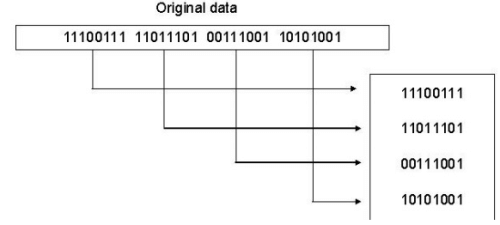Cyclic Redundancy Check
CRC is based on binary division. In this a sequence of redundant bits, called CRC remainder is appended to the end of a data unit so that the resulting data unit becomes exactly divisible by a second predetermined binary number. At its destination, the incoming data unit is divided by the same number. If at this step there is no reminder, the data unit is assumed to be intact and therefore accepted. A remainder indicates that the data unit has been changed in transit and therefore must be rejected.

Here, the remainder is the CRC. It must have exactly one less bit than the divisor, and appending it to the end of the data string must make the resulting bit sequence exactly divisible by the divisor.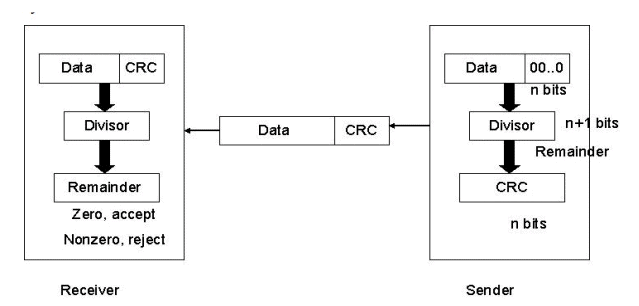First, a string of n-1 0s is appended to the data unit. The number of 0s is one less than the number of bits in the divisor which is n bits. Then the newly elongated data unit is divided by the divisor using a process called binary division. The remainder is CRC. The CRC is replaces the appended 0s at the end of the data unit.

The data unit arrives at the receiver first, followed by the CRC. The receiver treats whole string as the data unit and divides it by the same divisor that was used to find the CRC remainder. If the remainder is 0 then the data unit is error free. Otherwise it having some error and it must be discarded.

Checksum
The error detection method used by the higher layer protocols is called checksum. It consists of two arts. They are,
1.     checksum generator
2.     checksum checker

Checksum Generator:
In the sender, the checksum generator subdivides the data unit into equal segments of n bits. These segments are added with each other by using one‟s complement arithmetic in such a way that the total is also n bits long. That total is then complemented and appended to the end of the data unit.

Checksum Checker:
The receiver subdivides the data unit as above and adds all segments together and complements the result. If the extended data unit is intact, the total value found by adding the data segments and the checksum field should be zero. Otherwise the packet contains an error and the receiver rejects it.
Example
At the sender

Data unit: 10101001 00111001 10101001 00111001
Sum           1100010
Checksum  00011101

10101001 00111001 00011101
Sum           11111111
Complement         00000000
It means that the patter is ok.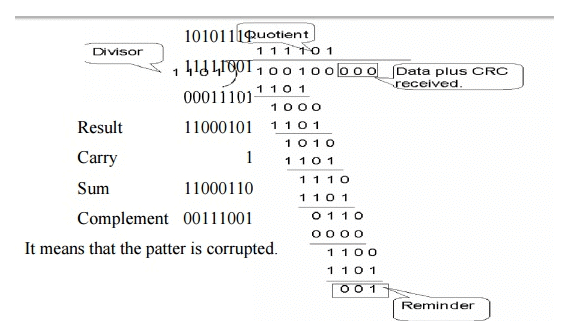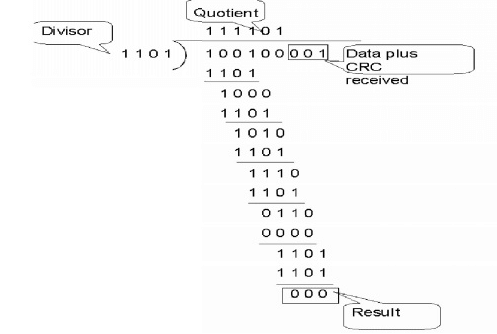2) Received data: 1010111 111001 00011101
10101111
11111001
00011101 Result 11000101 Carry 1 Sum 11000110 Complement 00111001
It means that the patter is corruptted.

Error Correction
Error correction is handled in two ways. In one, when an error is discovered, the receiver can have the sender retransmit the entire data unit. In the other, a receiver can use an error correcting code, which automatically corrects certain errors.

Types of error correction:
1.     Single bit error correction
2.     Burst bit error correction

Single Bit Error Correction
To correct a single bit error in an ASCII character, the error correction code must determine which of the seven bits has changed. In this case we have to determine eight different states: no error, error in position 1, error in position 2, error in position 3, error in position 4, error in position 5, error in position 6, error in position 7. It looks like a three bit redundancy code should be adequate because three bits can show eight different states. But what if an error occurs in the redundancy bits? Seven bits of data and three bits of redundancy bits equal 10 bits. So three bits are not adequate.

To calculate the number of redundancy bits (r) required to correct a given number of data bits (m) we must find a relationship between m and r.
If the total number of bits in a transmittable unit is m+r then r must be able to indicate at least m+r+1 different state. Of these, one state means no error and m+r states indicate the location of an error in each of the m+r positions.
So m+r+1 state must be discoverable by r bits. And r bits can indicate 2r different states. Therefore, 2rmust be equal to or greater than m+r+1;
2r >=m+r+1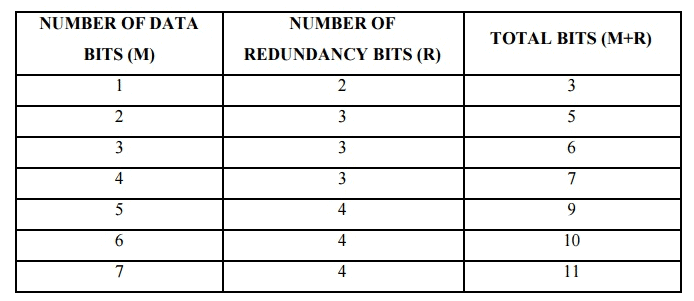Hamming Code:
The hamming code can be applied to data units of any length and uses the relationship between data and redundancy bits.
Positions of redundancy bits in hamming code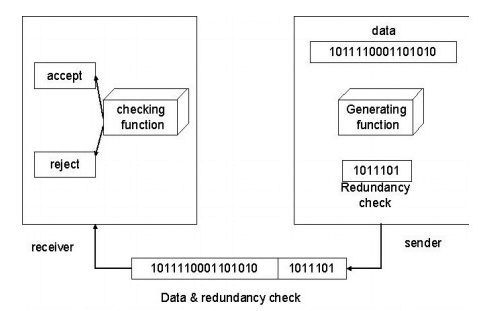The combinations used to calculate each of the four r values for a seven bit data sequence are as follows:
r1 :1,3,5,7,9,11
r2 : 2,3,6,7,10,11
r3 : 4,5,6,7
r4 : 8,9,10,11
Here, r1 bit is calculated using all bit positions whose binary representation includes a 1 in the rightmost position (0001, 0011, 0101, 0111, 1001, and 1011). The r2 bit is calculated using all bit positions with a 1 in the second position (0010, 0011, 0110, 0111, 1010 and 1011), and for r3 1 at third bit position (0100, 0101, 0110 and 0111) for r4 1 at fourth bit position (1000, 1001, 1010 and 1011).

Calculating the r Values:
In the first step, we place each bit of the original character in its appropriate positions in the 11 bit unit. Then, we calculate the even parities for the various bit combinations. The parity value of each combination is the value of the corresponding r bit. For example r1 is calculated to provide even parity for a combination of bits 3, 5, 7, 9, 11.

Error Detection and Correction:
Example:
At the sender:

Data to be sent: 1001101
Redundancy bit calculation: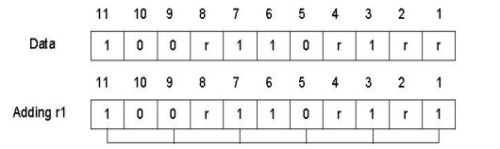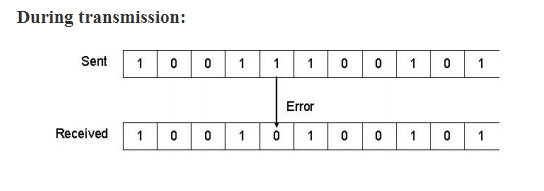Data sent with redundancy bits: 10011100101The receiver takes the transmission and recalculates four new r values using the same set of bits used by the sender plus the relevant parity (r) bit for each set. Then it assembles the new parity values into a binary number in order of r position (r8, r4, r2, r1).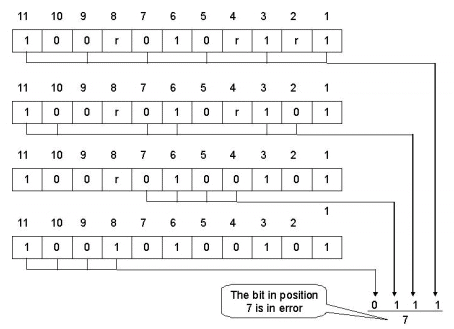Once the bit is identified, the receiver can reverse its value and correct the error.

Burst Bit Error Correction:
A hamming code can be designed to correct burst errors of certain length. The number of redundancy bits required to make these corrections, however, is dramatically higher than that required for single bit errors. To correct double bit errors, for example, we must take into consideration that the two bits can be a combination of any two bits in the entire sequence. Three bit correction means any three bits in the entire sequence and so on.

The document Error Detection & Correction: Its Types Notes | Study Computer Networks - Computer Science Engineering (CSE) is a part of the Computer Science Engineering (CSE) Course Computer Networks.
All you need of Computer Science Engineering (CSE) at this link: Computer Science Engineering (CSE)Use Code STAYHOME200 and get INR 200 additional OFF

## Computer Networks

12 videos|72 docs|53 tests

Track your progress, build streaks, highlight & save important lessons and more!

,

,

,

,

,

,

,

,

,

,

,

,

,

,

,

,

,

,

,

,

,

;